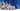# JavaScript 中 Array.from() 的五个有用的应用

JavaScript 中有一个这样的函数 `Array.from`：允许在 JavaScript 集合(数组、类数组对象、或者是字符串，map，set 等可迭代对象) 上进行有用的转换。

## 介绍

``Array.from(arrayLikeOrIterable[, mapFunction[, thisArg]])``
• `arrayLikeOrIterable`：必传参数，想要转换成数组的类数组对象可迭代对象
• `mapFunction`：可选参数，`mapFunction(item，index){...}` 是在集合中的每个项目上调用的函数。返回的值将插入到新集合中。
• `thisArg`：可选参数，执行回调函数 `mapFunction``this` 对象。这个参数很少使用。

``````const someNumbers = { 0: 10, 1: 15, length: 2 };

Array.from(someNumbers, (value) => value * 2); // => [20, 30]``````

## 将类数组转换成数组

`Array.from()` 的第一个用途是将类数组对象转换成数组。

``````function sumArguments() {
return Array.from(arguments).reduce((sum, num) => sum + num);
}

sumArguments(1, 2, 3); // => 6``````

`Array.from(arguments)` 将类数组对象 `arguments` 转换成一个数组，然后使用数组的 `reduce` 方法求和。

``````Array.from('Hey'); // => ['H', 'e', 'y']
Array.from(new Set(['one', 'two'])); // => ['one', 'two']

const map = new Map();
map.set('one', 1);
map.set('two', 2);
Array.from(map); // => [['one', 1], ['two', 2]]``````

## 克隆一个数组

``````const numbers = [3, 6, 9];
const numbersCopy = Array.from(numbers);

numbers === numbersCopy; // => false``````

`Array.from(numbers)` 创建了对 `numbers` 数组的浅拷贝，`numbers === numbersCopy` 的结果是 `false`，意味着虽然 `numbers``numbersCopy` 有着相同的项，但是它们是不同的数组对象。

``````function recursiveClone(val) {
return Array.isArray(val) ? Array.from(val, recursiveClone) : val;
}

const numbers = [
[0, 1, 2],
['one', 'two', 'three'],
];
const numbersClone = recursiveClone(numbers);

numbersClone; // => [[0, 1, 2], ['one', 'two', 'three']]
numbers === numbersClone; // => false``````

`recursiveClone()` 能够对数组的深拷贝，通过判断 数组的 item 是否是一个数组，如果是数组，就继续调用 `recursiveClone()` 来实现了对数组的深拷贝。

## 使用值填充数组

``````const length = 3;
const init = 0;
const result = Array.from({ length }, () => init);

result; // => [0, 0, 0]``````

`result` 是一个新的数组，它的长度为 3，数组的每一项都是 0。调用 `Array.from()` 方法，传入一个类数组对象 `{ length }` 和 返回初始化值的 `mapFunction` 函数。

``````const length = 3;
const init = 0;
const result = Array(length).fill(init);

fillArray2(0, 3); // => [0, 0, 0]``````

`fill()` 使用初始值正确填充数组。

### 使用对象填充数组

``````const length = 3;
const resultA = Array.from({ length }, () => ({}));
const resultB = Array(length).fill({});

resultA; // => [{}, {}, {}]
resultB; // => [{}, {}, {}]

resultA === resultA; // => false
resultB === resultB; // => true``````

`Array.from()` 返回的 `resultA` 使用不同空对象实例进行初始化。之所以发生这种情况是因为每次调用时，`mapFunction`，即此处的 `() => ({})` 都会返回一个新的对象。

### 使用 array.map 怎么样？

``````const length = 3;
const init = 0;
const result = Array(length).map(() => init);

result; // => [undefined, undefined, undefined]``````

`map()` 方法似乎不正常，创建出来的数组不是预期的 `[0, 0, 0]`，而是一个有 3 个空项的数组。

## 生成数字范围

``````function range(end) {
return Array.from({ length: end }, (_, index) => index);
}

range(4); // => [0, 1, 2, 3]``````

`range()` 函数中，`Array.from()` 提供了类似数组的 `{length：end}`，以及一个简单地返回当前索引的 `map` 函数 。这样你就可以生成值范围。

## 数组去重

``````function unique(array) {
return Array.from(new Set(array));
}

unique([1, 1, 2, 3, 3]); // => [1, 2, 3]``````

## 结论

`Array.from()` 方法接受类数组对象以及可迭代对象，它可以接受一个 map 函数，并且，这个 `map` 函数不会跳过值为 `undefined` 的数值项。这些特性给 `Array.from()` 提供了很多可能。